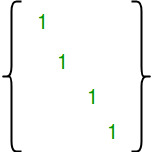Related Articles
Number of Reflexive Relations on a Set
• Difficulty Level : Easy
• Last Updated : 18 Apr, 2018

Given a number n, find out number of Reflexive Relation on a set of first n natural numbers {1, 2, ..n}.

Examples :

```Input  : n = 2
Output : 4
The given set A = {1, 2}. The following
are reflexive relations on A * A :
{{1, 1), (2, 2)}
{(1, 1), (2, 2), (1, 2)}
{(1, 1), (2, 2), (1, 2), (2, 1)}
{(1, 1), (2, 2), (2, 1)}

Input  : n = 3
Output : 64
The given set is {1, 2, 3}. There are
64 reflexive relations on A * A :
```

Explanation :

Reflexive Relation : A Relation R on A a set A is said to be Reflexive if xRx for every element of x ? A.

The number of reflexive relations on an n-element set is 2n2 – n

How does this formula work?
A relation R is reflexive if the matrix diagonal elements are 1.If we take a closer look the matrix, we can notice that the size of matrix is n2. The n diagonal entries are fixed. For remaining n2 – n entries, we have choice to either fill 0 or 1. So there are total 2n2 – n ways of filling the matrix.

## CPP

 `// C++ Program to count reflexive relations ` `// on a set of first n natural numbers. ` `#include ` `using` `namespace` `std; ` ` `  `int` `countReflexive(``int` `n) ` `{ ` `   ``// Return 2^(n*n - n)  ` `   ``return` `(1 << (n*n - n)); ` `} ` ` `  `int` `main() ` `{   ` `    ``int` `n = 3; ` `    ``cout << countReflexive(n); ` `    ``return` `0; ` `} `

## Java

 `// Java Program to count reflexive ` `// relations on a set of first n ` `// natural numbers. ` ` `  `import` `java.io.*; ` `import` `java.util.*; ` ` `  `class` `GFG { ` `     `  `static` `int` `countReflexive(``int` `n) ` `{ ` ` `  `// Return 2^(n*n - n)  ` `return` `(``1` `<< (n*n - n)); ` ` `  `} ` ` `  `// Driver function ` `    ``public` `static` `void` `main (String[] args) { ` `    ``int` `n = ``3``; ` `    ``System.out.println(countReflexive(n)); ` `         `  `    ``} ` `} ` ` `  `// This code is contributed by Gitanjali. `

## Python3

 `# Python3 Program to count ` `# reflexive relations ` `# on a set of first n ` `# natural numbers. ` ` `  `  `  `def` `countReflexive(n): ` ` `  `    ``# Return 2^(n*n - n)  ` `    ``return` `(``1` `<< (n``*``n ``-` `n)); ` ` `  `# driver function ` `n ``=` `3` `ans ``=` `countReflexive(n); ` `print` `(ans) ` ` `  `# This code is contributed by saloni1297 `

## C#

 `// C# Program to count reflexive ` `// relations on a set of first n ` `// natural numbers. ` `using` `System; ` ` `  `class` `GFG { ` `     `  `    ``static` `int` `countReflexive(``int` `n) ` `    ``{ ` `     `  `        ``// Return 2^(n*n - n)  ` `        ``return` `(1 << (n*n - n)); ` `         `  `    ``} ` `     `  `    ``// Driver function ` `    ``public` `static` `void` `Main () { ` `         `  `    ``int` `n = 3; ` `    ``Console.WriteLine(countReflexive(n)); ` `    ``} ` `} ` ` `  `// This code is contributed by vt_m. `

## PHP

 ` `

Output :

```64
```

Attention reader! Don’t stop learning now. Get hold of all the important DSA concepts with the DSA Self Paced Course at a student-friendly price and become industry ready.

My Personal Notes arrow_drop_up
Recommended Articles
Page :